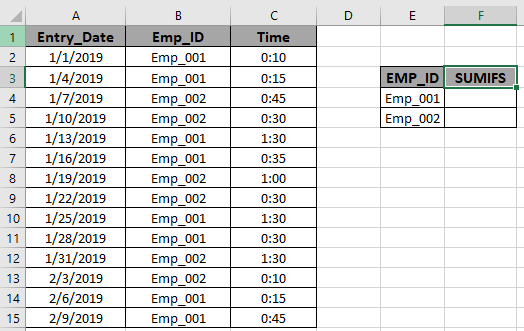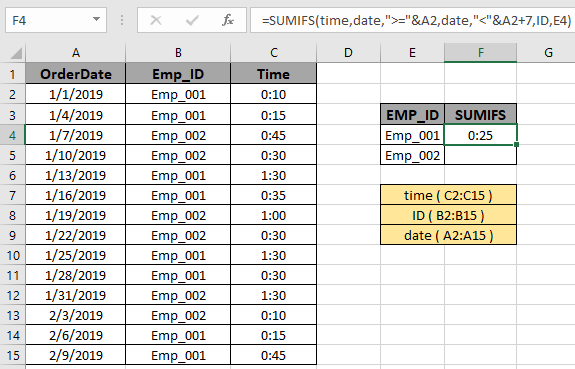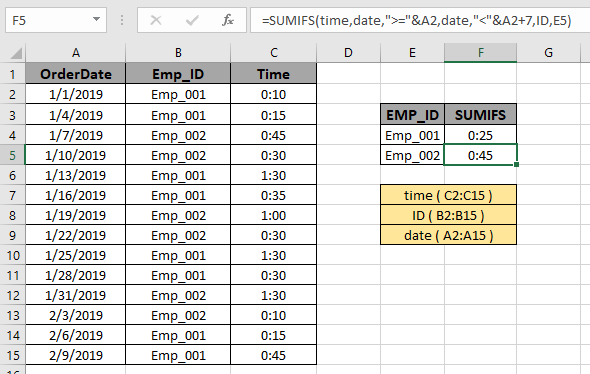# Find the SUM time values having multiple criteria

In this article, we will learn about how to Sum if values having multiple criteria using SUMIFS in Excel.

In simple words, while working with long data sheet. Sometimes we need to find the find the sum of time values having multiple criteria. SUMIFS function formula finds the SUM of the array where multiple conditions needs to be satisfied. So we made a formula out of the SUMIFS function to meet multiple conditions.

SUMIFS function returns the sum of range having multiple criterias.

Generic formula:

= SUMIFS ( Sum_range , range1 , "value1" , range2, ">value2", ….)

Variables:

Sum_range : range ( time values ) where sum is required.

range1 : range where criteria value1 is applied.

Value1 : value criteria applied on range1.

range2 : range where criteria value2 is applied.

Value2 : value criteria applied on range2.

EXAMPLE:

In a database entry of employees is recorded with time. Now the company wants to know how much time a particular employee spends time.We need to get the SUM of time value having 2 conditions. Conditions are in following statements.

1. Date must be after the January 1st, 2019.
2. Date must be before the January 7th, 2019.
3. Matching Employee ID for particular employee.

So we will use the formula to get the total time

= SUMIFS ( time , date , ">=" & A2 , date , "<" & A2+7, ID , E4)

time ( C2:C15 ) : Sum_range where values gets sum

ID ( B2:B15 ) :  range where criteria is applied.

date , ">=" & A2 : Dates after the January 1st, 2019.

date , "<" & A2+7 : Dates before the January 7th, 2019.

Explanation for the formula:

Here the SUMIFS function satisfies all the conditions mentioned. The function returns the SUM of the time values only which satisfies all the criteria in the formula.Here the arguments to the function is given as cell references and named ranges. The yellow marked boxes indicates the named ranges used.
As you can see the formula returns 0:25 , the Total time spent by Emp_001.

Now to calculate the time spent by Emp_002. We will use the formula:

= SUMIFS ( time , date , ">=" & A2 , date , "<" & A2+7, ID , E5)As you can see the formula returns 0:45 , the Total time spent by Emp_002.

Here are some observational notes when using the formula.
Notes:

1. The SUMIFS function supports logical operators like <, >, <>, = but these are used using double quote sign ( " ) .
2. The SUMIFS function also supports Wildcards ( * , ? ) which helps in extracting values having phrases.
3. Non - numeric values must be provided in double quotes ("value").
4. The function returns the sum of the values satisfying all the conditions.

Hope you understood how to Find the SUM time values having multiple criteria in Excel. Explore more articles on Excel SUMIFS function here. Please feel free to state your query or feedback for the above article.

Related Articles

3 SUMIF with Or Formulas : multiple use of SUMIF function with a logic OR function in excel.

How to Use SUMIFS Function in Excel : Use of SUMIFS function explained with example in excel.

SUMIFS using AND-OR logic : Use of SUMIFS function with 2 logic OR & AND function in excel.

SUMIF with non-blank cells : Use of SUMIF function to catch blank cells in Excel.

SUMIFS with dates in Excel : Use of SUMIFS function with dates as criteria in Excel.

Popular Articles

Edit a dropdown list

If with conditional formatting

If with wildcards

Vlookup by date

Terms and Conditions of use

The applications/code on this site are distributed as is and without warranties or liability. In no event shall the owner of the copyrights, or the authors of the applications/code be liable for any loss of profit, any problems or any damage resulting from the use or evaluation of the applications/code.